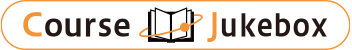### CourseDetailDegree
Bachelor
1
Course delivery methods
Subject
Physical sciences, Mathematical sciences, Engineering & technology
Program
School
School of Science and Engineering
Department
Bachelor's Program in Interdisciplinary Engineering
Campus
Tsukuba Campus
Classroom
3A213
Course Offering Year
2023-2024
Course Offering Month
October - February
Weekday and Period
Wed4,5
Capacity
Credits
3.0
Language
English
Course Number
FJ20004

### Linear Algebra I University of Tsukuba

#### Course Overview

This course introduces the basic ideas of vector, matrix and their operations and how to solve linear equations using matrices and vectors. The primary goal of this course is to understand the systems of linear equations, classifications of matrices and their applications. Although most of the problems can be solved without Mathematica, you are encouraged to solve the homework using the software once you know how to solve the problems. The course is a prerequisite for “Linear Algebra II”

#### Learning Achievement

This course introduces the basic ideas of vector, matrix and their operations and how to solve linear equations using matrices and vectors. The primary goal of this course is to understand the systems of linear equations, classifications of matrices and their applications. Although most of the problems can be solved without Mathematica, you are encouraged to solve the homework using the software once you know how to solve the problems. The course is a prerequisite for “Linear Algebra II”

#### Competence

Related to 1. Mathematical logic and calculation skills.

#### Course prerequisites

Math B at high school in Japan.

Quiz + homework 30%
Midterm Exam 35 %
Final Exam 35%

#### Course schedule

Introduce vectors and its operation
Introduce matrix and related matrix ？vector to linear equation
The idea of elimination and elimination using matrices
Rules of Matrix Operations and Inverse of Matrices
Review Inverse of Matrices and Exercises
Elimination (Factorization:) A=LU
Transpose and Permutations
Review and midterm examination
Answer to midterm exam and Spaces of Vectors
The Null spaces of A
The Complete Solution to Ax=b + review
Independence, and Dimension of the Four subspaces
Orthogonality of the Four Subspaces and Projections
Least squares approximations and Orthonormal Bases
Applications: Graphs and Networks + Review

#### Course type

Lectures and Class Exercises

Tong Xiao-Min

#### Other information

*You are encouraged to discuss with others about the contents of the course and homework, but you must work out the homework yourself. No late homework will be accepted.
* All exams are closed book and you cannot use notes, calculators during the exams.

#### Site for Inquiry

Link to the syllabus provided by the university